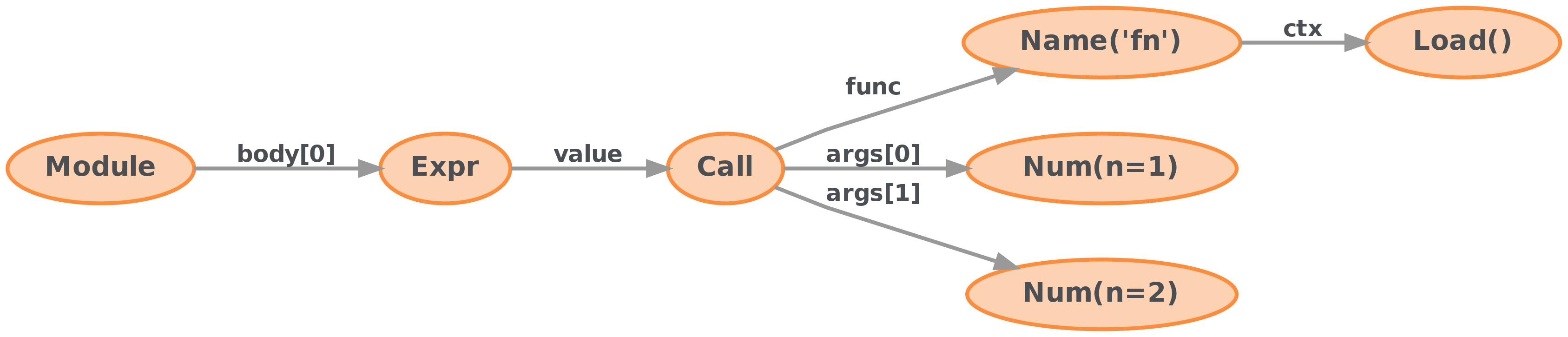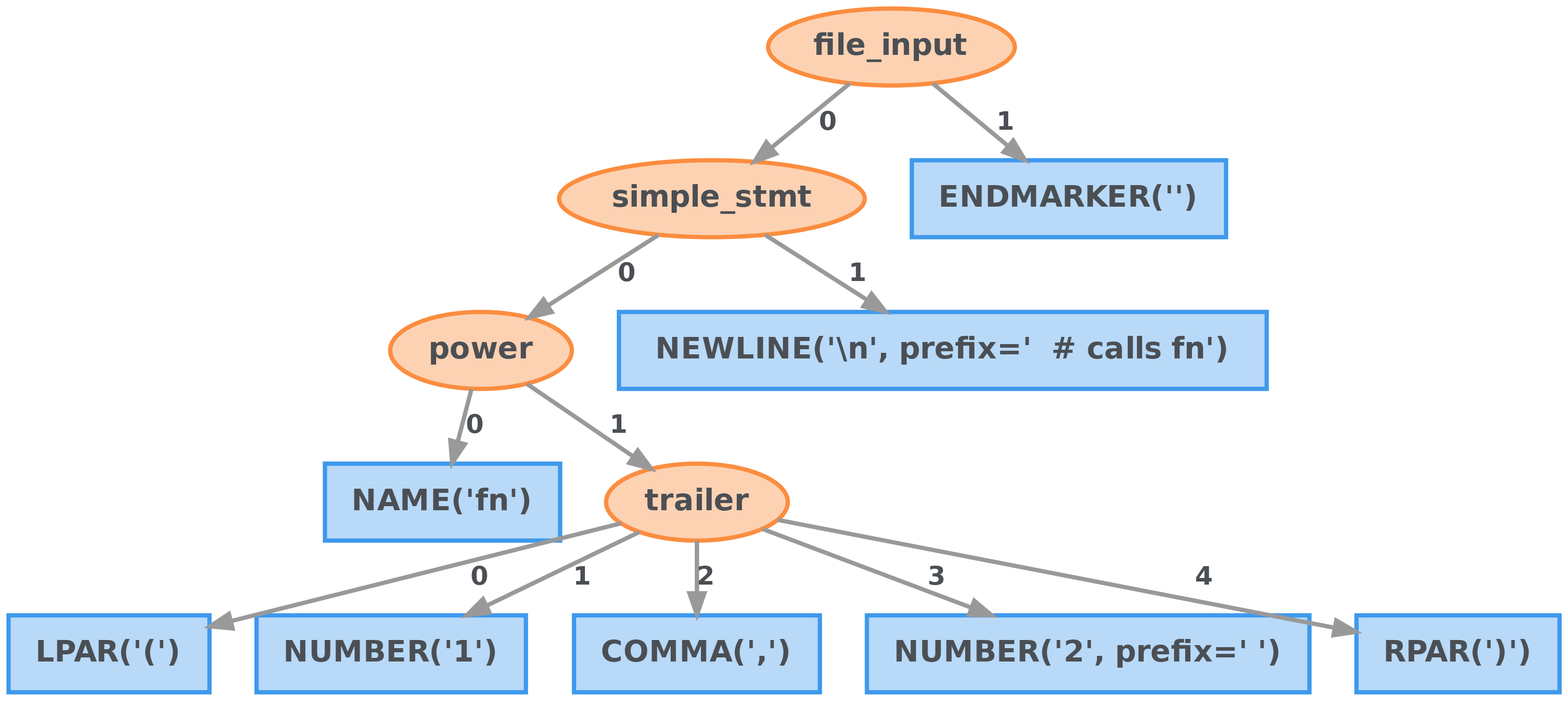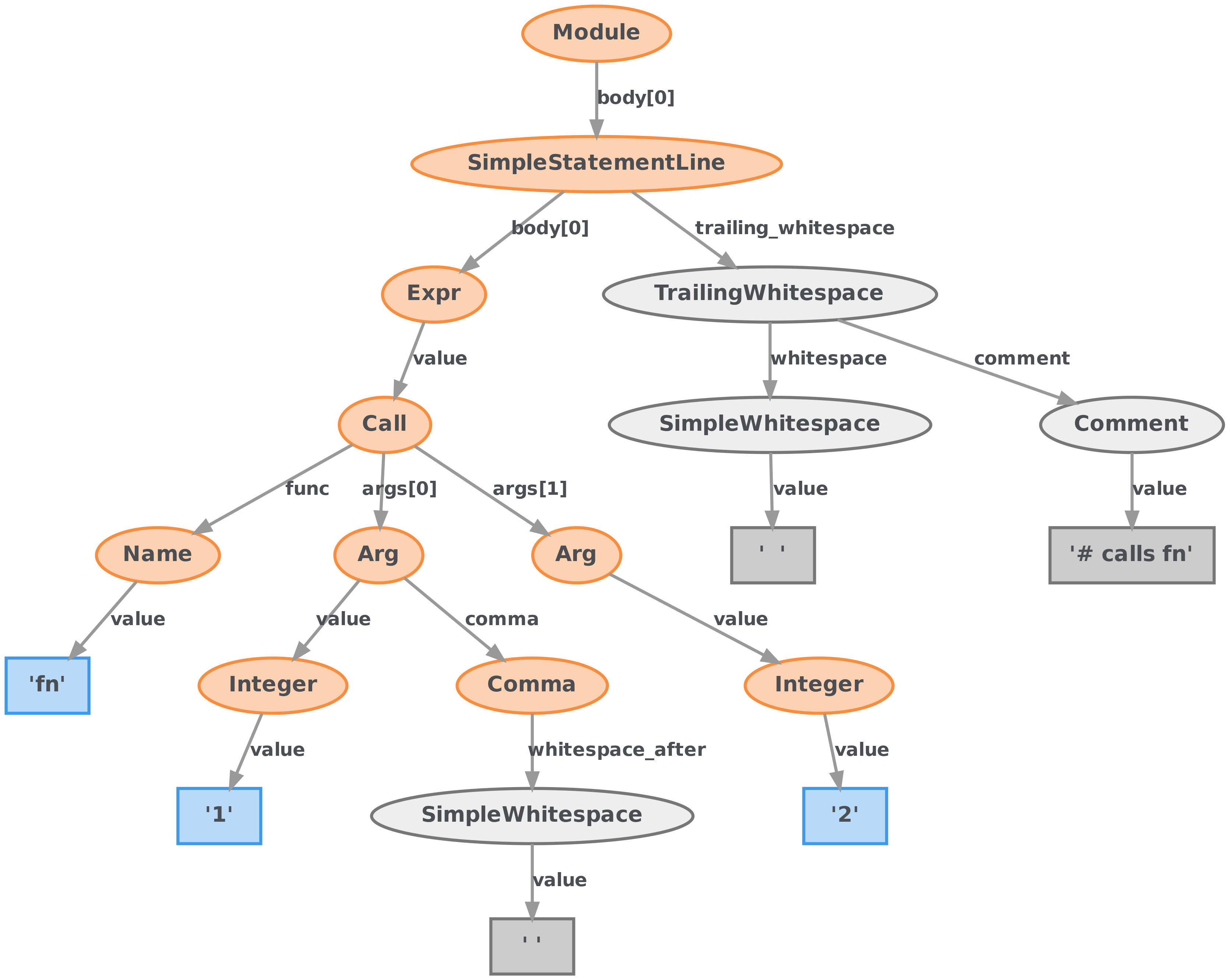# Why LibCST?¶

Python’s ast module already provides a syntax tree. Why do we need another?

LibCST creates a compromise between an Abstract Syntax Tree (AST) and a traditional Concrete Syntax Tree (CST). By carefully reorganizing and naming node types and fields, we’ve created a lossless CST that looks and feels like an AST.

## Abstract Syntax Trees (AST)¶

Let’s look at Python’s AST for the following code snippet:

```fn(1, 2)  # calls fn
```
```ast.Module(
body=[
ast.Expr(
value=ast.Call(
args=[ast.Num(n=1), ast.Num(n=2)],
keywords=[],
),
),
],
)
```This syntax tree does a great job of preserving the semantics of the original code, and the structure of the tree is relatively simple.

However, given only the AST, it wouldn’t be possible to reprint the original source code. Like a JPEG, the Abstract Syntax Tree is lossy.

• The comment we left at the line is gone.

• There’s a newline at the end of the file, but the AST doesn’t tell us that. It also doesn’t tell us if it’s `\n`, `\r`, or `\r\n`.

• We’ve lost some information about the whitespace between the first and second argument.

Abstract Syntax Trees are good for tools like compilers and type checkers where the semantics of code is important, but the exact syntax isn’t.

## Concrete Syntax Trees (CST)¶

A popular CST library for Python is lib2to3, which powers tools like 2to3 and Black. Let’s look at the syntax tree it generates for the same piece of code:

```fn(1, 2)  # calls fn
```
```Node(
file_input,
children=[
Node(
simple_stmt,
children=[
Node(
power,
children=[
Leaf(NAME, "fn", prefix=""),
Node(
trailer,
children=[
Leaf(LPAR, "(", prefix=""),
Node(
arglist,
children=[
Leaf(NUMBER, "1", prefix=""),
Leaf(COMMA, ",", prefix=""),
Leaf(NUMBER, "2", prefix=" "),
],
),
Leaf(RPAR, ")", prefix=""),
],
),
],
),
Leaf(
NEWLINE,
"\n",
prefix="  # calls fn",
),
],
prefix=""
),
Leaf(ENDMARKER, "", prefix=""),
],
prefix="",
)
```This tree is lossless. It retains enough information to reprint the exact input code by storing whitespace information in `prefix` properties. This makes it a “Concrete” Syntax Tree, or CST.

However, much of the semantics of the code is now difficult to understand and extract. lib2to3 presents a tree that closely matches Python’s grammar which can be hard to manipulate for complex operations.

• Adding or removing a parameter from `fn` requires careful preservation of `COMMA` nodes.

• Whitespace and comment ownership is unclear. Deleting nodes could result in invalid generated code.

Concrete Syntax Trees are good for operations that don’t significantly change the tree and tools that do not wish to change the semantics of the code itself, such as Black.

## LibCST¶

LibCST takes a compromise between the two formats outlined above. Like a CST, LibCST preserves all whitespace and can be reprinted exactly. Like an AST, LibCST parses source into nodes that represent the semantics of the code.

```fn(1, 2)  # calls fn
```
```Module(
body=[
SimpleStatementLine(
body=[
Expr(
value=Call(
func=Name(
value='fn',
lpar=[],
rpar=[],
),
args=[
Arg(
value=Integer(
value='1',
lpar=[],
rpar=[],
),
keyword=None,
equal=MaybeSentinel.DEFAULT,
comma=Comma(
whitespace_before=SimpleWhitespace(
value='',
),
whitespace_after=SimpleWhitespace(
value=' ',
),
),
star='',
whitespace_after_star=SimpleWhitespace(
value='',
),
whitespace_after_arg=SimpleWhitespace(
value='',
),
),
Arg(
value=Integer(
value='2',
lpar=[],
rpar=[],
),
keyword=None,
equal=MaybeSentinel.DEFAULT,
comma=MaybeSentinel.DEFAULT,
star='',
whitespace_after_star=SimpleWhitespace(
value='',
),
whitespace_after_arg=SimpleWhitespace(
value='',
),
),
],
lpar=[],
rpar=[],
whitespace_after_func=SimpleWhitespace(
value='',
),
whitespace_before_args=SimpleWhitespace(
value='',
),
),
semicolon=MaybeSentinel.DEFAULT,
),
],
trailing_whitespace=TrailingWhitespace(
whitespace=SimpleWhitespace(
value='  ',
),
comment=Comment(
value='# calls fn',
),
newline=Newline(
value=None,
),
),
),
],
footer=[],
encoding='utf-8',
default_indent='    ',
default_newline='\n',
has_trailing_newline=True,
)
```LibCST preserves whitespace by parsing it using an internal whitespace parser and assigning it to relevant nodes. This allows for much more granular whitespace ownership and greatly reduces the amount of work necessary to perform complex manipulations. Additionally, it is fully typed. A node’s children are well-defined and match the semantics of Python.

However, this does come with some downsides.

• It is more difficult to implement tools that focus almost exclusively on whitespace on top of LibCST instead of lib2to3. For example, Black would need to modify whitespace nodes instead of prefix strings, making its implementation much more complex.

• The equivalent AST for a Python module will usually be simpler. We must preserve whitespace ownership by assigning it to nodes that make the most sense which requires us to introduce nodes such as `Comma`.

• Parsing with LibCST will always be slower than Python’s AST due to the extra work needed to assign whitespace correctly.

Nevertheless, we think that the trade-offs made in LibCST are worthwhile and offer a great deal of flexibility and power.